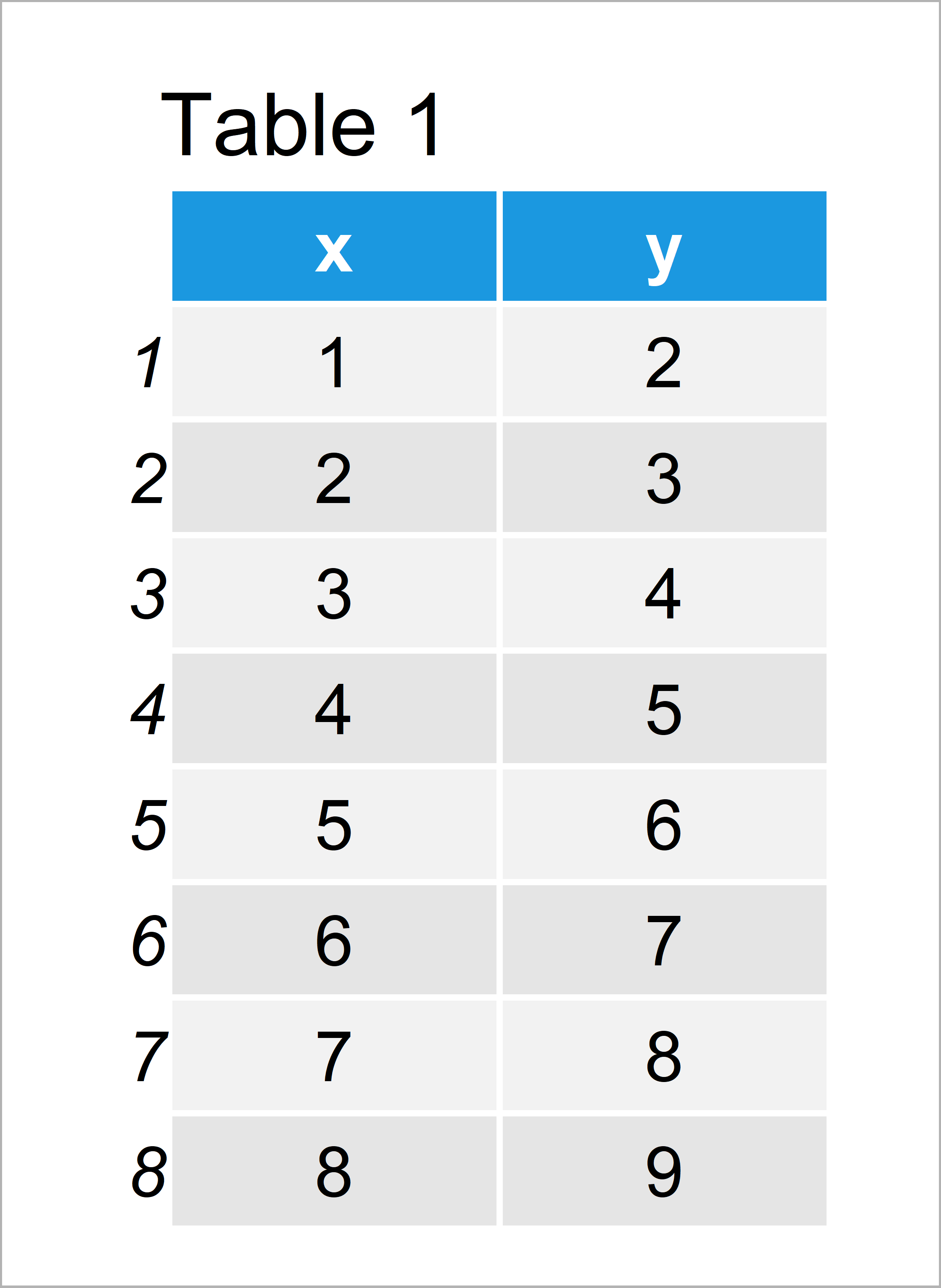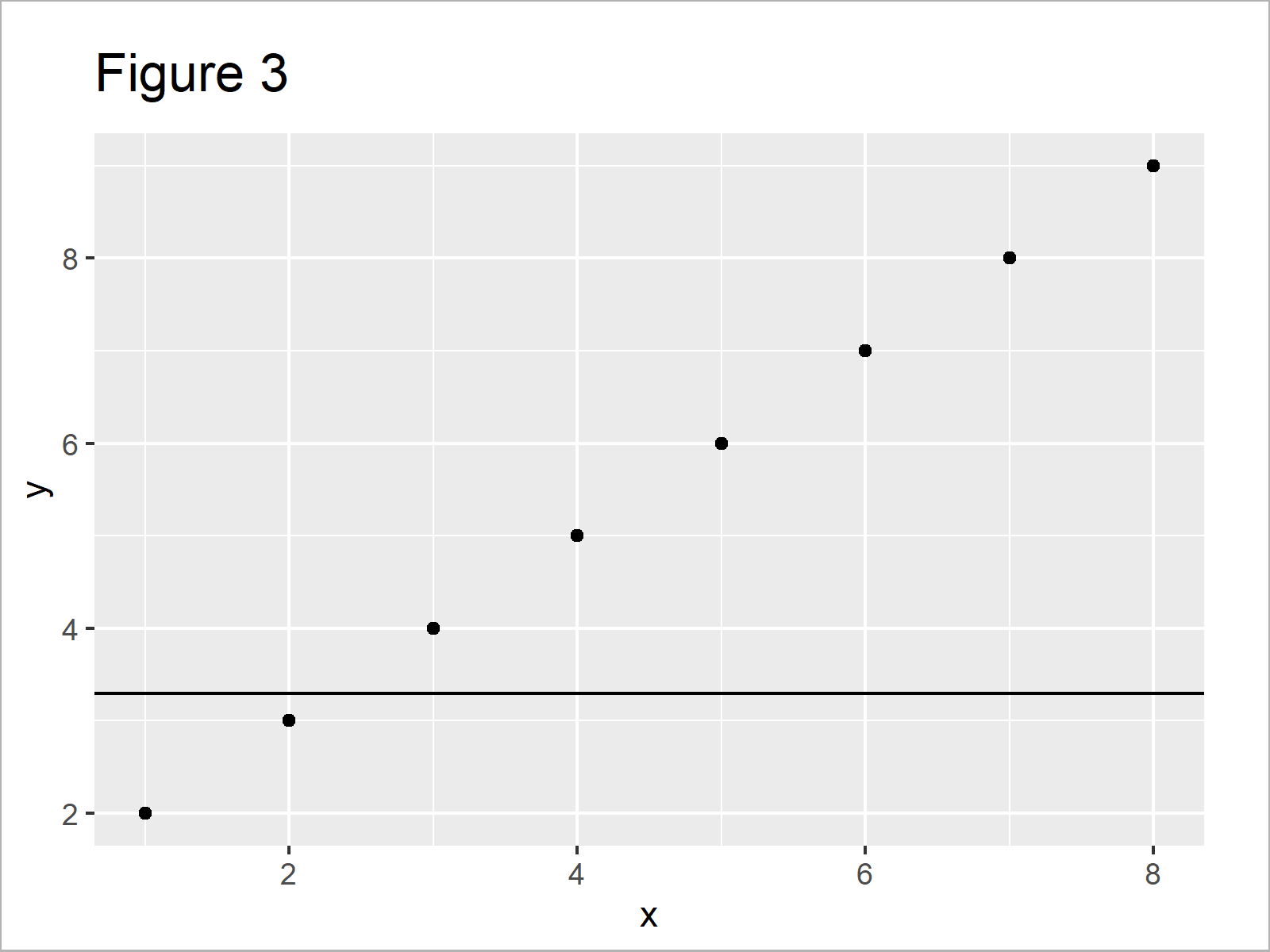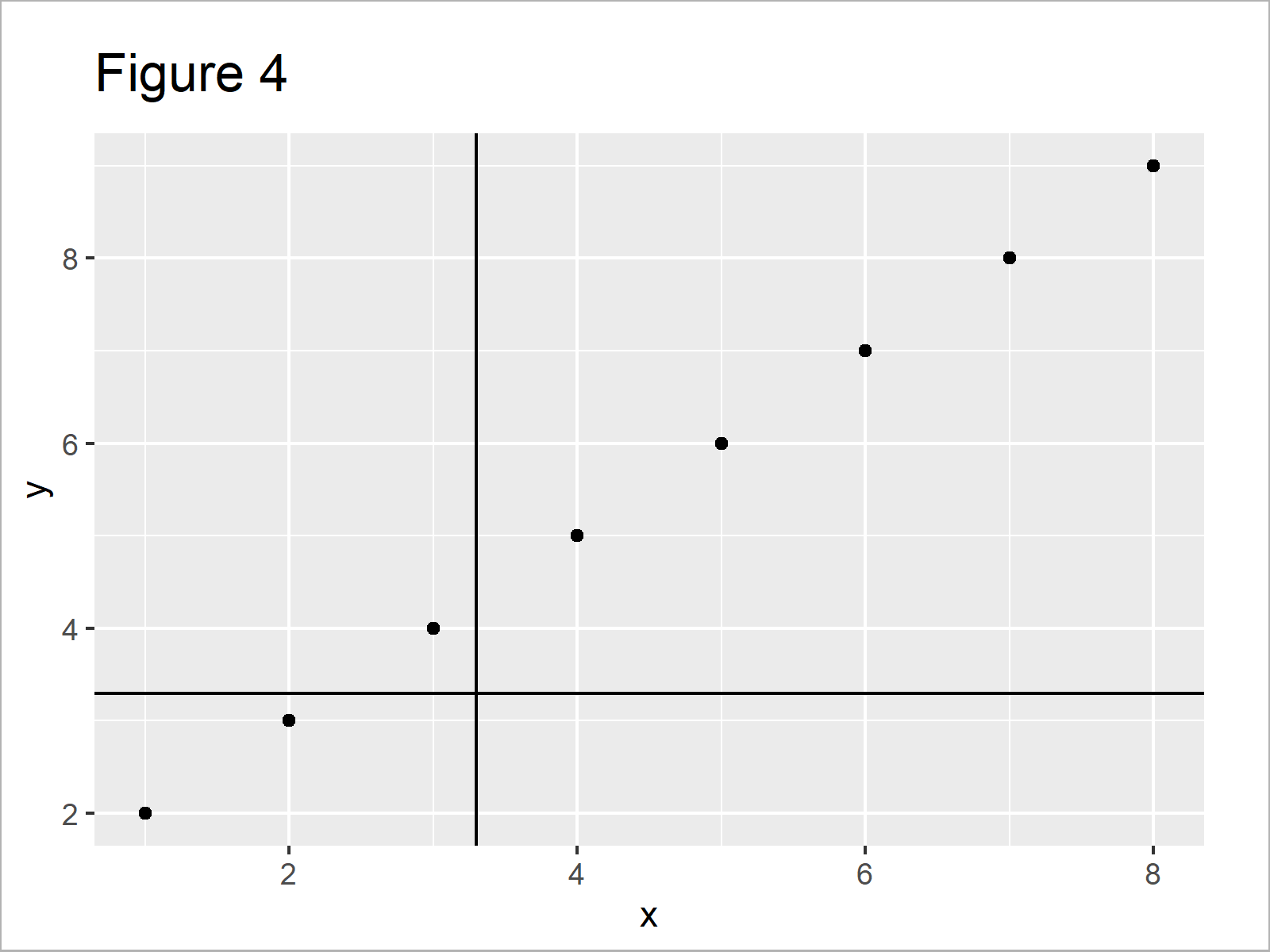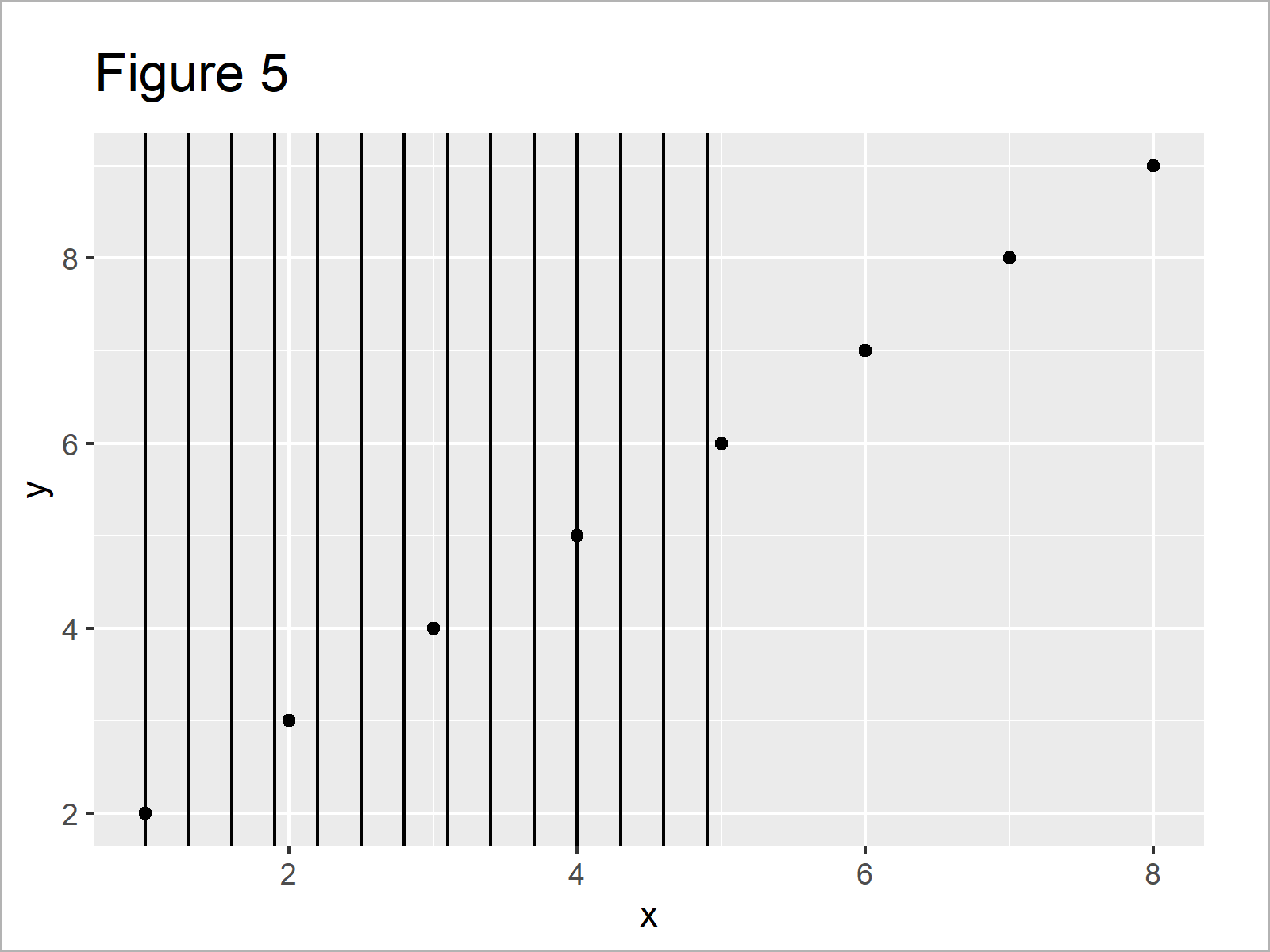# Add Vertical & Horizontal Line to gglot2 Plot in R (4 Examples)

In this tutorial you’ll learn how to draw vertical and horizontal lines to a ggplot2 graph in R programming.

The article will consist of four examples for the drawing of lines. More precisely, the tutorial consists of these contents:

Let’s get started:

## Example Data, Packages & Default Graphic

I use the following data as basement for this R tutorial:

```data <- data.frame(x = 1:8,         # Create example data
y = 2:9)
data                                # Print example data```Table 1 shows the structure of the example data: It consists of eight rows and two columns.

We also have to install and load the ggplot2 package, to be able to use the corresponding functions and commands.

```install.packages("ggplot2")         # Install ggplot2 package

Now, we can plot the data without any lines as follows:

```ggp <- ggplot(data, aes(x, y)) +    # Create ggplot2 plot
geom_point()
ggp                                 # Draw ggplot2 plot```In Figure 1 it is shown that we have drawn a ggplot2 scatterplot by executing the previous R code.

## Example 1: Add Vertical Line to ggplot2 Plot Using geom_vline() Function

In this example, I’ll explain how to print a vertical line to a ggplot2 plot.

For this, we can use the geom_vline function and the xintercept argument:

```ggp +                               # Draw line to plot
geom_vline(xintercept = 3.3)```In Figure 2 it is shown that we have created a scatterplot with a vertical line at the x-axis position 3.3.

## Example 2: Add Horizontal Line to ggplot2 Plot Using geom_hline() Function

Example 2 explains how to draw a horizontal line using the geom_hline function and the yintercept argument.

```ggp +                               # Draw line to plot
geom_hline(yintercept = 3.3)```In Figure 3 it is shown that we have added a vertical line to our plot using the previous R code.

## Example 3: Add Vertical & Horizontal Lines to ggplot2 Plot

In Example 3, I’ll show how to add vertical and horizontal lines to the same ggplot2 graph.

```ggp +                               # Draw line to plot
geom_vline(xintercept = 3.3) +
geom_hline(yintercept = 3.3)```As shown in Figure 4, we have plotted two lines to our plot.

## Example 4: Add Multiple Vertical Lines to ggplot2 Plot Using seq() Function

This example shows how to add a sequence of multiple lines using the seq function.

```ggp +                               # Draw line to plot
geom_vline(xintercept = seq(1, 5, by = 0.3))```By running the previous syntax we have plotted Figure 5, i.e. a scatterplot with a sequence of vertical lines.

## Video & Further Resources

Would you like to know more about ggplot2 graphics? Then you could have a look at the following video instruction of my YouTube channel. I’m explaining the contents of this article in the video.

Please accept YouTube cookies to play this video. By accepting you will be accessing content from YouTube, a service provided by an external third party.If you accept this notice, your choice will be saved and the page will refresh.

In addition, you may want to have a look at some of the related articles on my homepage. A selection of articles about graphics in R is listed below.

This article has illustrated how to add a line to a ggplot2 graphic in the R programming language. Please let me know in the comments section, if you have any further questions or comments.

Subscribe to the Statistics Globe Newsletter